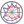Course title
Simulation Engineering
 YAMAZAWA HiroshiCourse description
The aim of the course are to understand the root of the problems of population explosion · population prediction · growth limit, propagation · infection of rhyming vocabulary, food chain as a mathematical model and learn meaning of prescribing mathematical prediction result. We would study the simulation language based on the mathematical programming method, which is indispensable for managing the social / economic system and production system.
Purpose of class
To model the phenomena of society as the mathematical modeling. Simulation is indispensable for understanding complex social and economic systems and production system management. In the lecture, we would conduct the planning, analysis and evaluation of mathematical models as a part and learn management method of production system using simulation language based on mathematical programming method.
Goals and objectives
1. To understand the necessity to change the operation procedure automatically according to the change of the environmental condition and the design to operate at the optimum condition each time.
2. To learn methodology of social phenomena from construction, analysis and evaluation of mathematical models of social phenomena.
3. To study the simulation language to manage the production system.
Language
Japanese
Class schedule

Class schedule HW assignments (Including preparation and review of the class.) Amount of Time Required
1. Introduction: Preparation of mathematics and meaning of 'prediction' and simulation as methodology. To confirm the basis of differentiation, integration and differential equation. 50minutes
2. To construct a model describing population increase and population explosion, to analyze and evaluate its mathematical model. To review differential equations and organize equations corresponding to various conservation laws. 50minutes
3. To study modeling that prevent population explosion and understand what kind of parameters are required. To review differential equations and organize equations corresponding to various conservation laws. 50minutes
4. To construct and analyze a mathematical model describing the mechanism its changes the characteristics of the whole system such as propagation of rumors vulgarism in social system, and to evaluate its meaning. To review differential equations and organize equations corresponding to various conservation laws. 50minutes
5. To construct and analyze a mathematical model on food chain in social system, and to evaluate the result and understand the characteristics of the system in a chain relationship. Several kinds of inequalities appear when predicting only by differentiation or information change. To organize the solution for solve the conditional differential equation. 50minutes
6. To learn general methods for constructing mathematical models of various social systems and methods for inferring respective system characteristics. To organize the equations corresponding to various conservation laws. 50minutes
7. Simulation language based on mathematical programming. To review linear mathematical programming method. 50minutes
8. Model of production made up of multiple production lines. To review linear mathematical programming method. 50minutes
9. Generalized model of production system and its characteristics. To review linear mathematical programming method. 50minutes
10. Miniaturization of large scale production plan and its meaning. To learn the tasks and mathematical models in planning the production plan. 50minutes
11. Solutions for large scale production plan and its meaning. To learn the tasks and mathematical models in planning the production plan. 50minutes
12. Change of production plan by sensitive analysis and its mathematical model. To learn the meaning of sensitive analysis. 50minutes
13. Production planning example using simulation language - 1 To learn the superiority of simulation languages from application to concrete tasks. 50minutes
14. Production planning example using simulation language - 2 To learn the superiority of simulation languages from application to concrete tasks. 50minutes
Total. - - 700minutes
Relationship between 'Goals and Objectives' and 'Course Outcomes'

Analysis of mathematical model Total.
1. 20% 20%
2. 20% 20%
3. 20% 20%
4. 20% 20%
5. 20% 20%
Total. 100% -
Evaluation method and criteria
5-6 reports.
Textbooks and reference materials
Will be introduced in the class
Prerequisites
We will recommend to prepare by reading the introductory book of mathematical model.
Office hours and How to contact professors for questions
• On Monday.
Regionally-oriented
Non-regionally-oriented course
Development of social and professional independence
• Course that cultivates a basic problem-solving skills
Active-learning course
Most classes are interactive
Course by professor with work experience
Work experience Work experience and relevance to the course content if applicable
N/A N/A
Education related SDGs:the Sustainable Development Goals• 4.QUALITY EDUCATION